Courses

# Test: Forecasting Level - 2

## 10 Questions MCQ Test Engineering Mechanics | Test: Forecasting Level - 2

Description
This mock test of Test: Forecasting Level - 2 for Mechanical Engineering helps you for every Mechanical Engineering entrance exam. This contains 10 Multiple Choice Questions for Mechanical Engineering Test: Forecasting Level - 2 (mcq) to study with solutions a complete question bank. The solved questions answers in this Test: Forecasting Level - 2 quiz give you a good mix of easy questions and tough questions. Mechanical Engineering students definitely take this Test: Forecasting Level - 2 exercise for a better result in the exam. You can find other Test: Forecasting Level - 2 extra questions, long questions & short questions for Mechanical Engineering on EduRev as well by searching above.
QUESTION: 1

### Demand for the month of January is given as 200; February 50; March 150. If a 9 month moving average method is used for forecasting and the forecasted value for the month of January is 100 units, then the forecast for the month of March using Exponential smoothening method is ___________Ans~ B

Solution: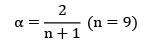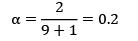FFeb = FJan + α(DJan − FJan ) = 100 + (200 − 100) × 0.2 = 120

Fmar = FFeb + α ∙ (DFeb − FFeb) = 120 + 0.2(50 − 120)

Fmar = 106

*Answer can only contain numeric values
QUESTION: 2

### A hospital has used a 9-month moving average forecasting method to forecast drug and dressing inventory requirements. The actual demand for one item is as shown in the following table. Using previous moving average data, convert to an exponential forecast and find the forecast for the month 33.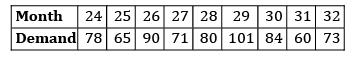Solution:

9 month moving average

Ft+1 = α ∙ Dt + α(1 − α) ∙ Dt−1 + α(1 − α)2 ∙ Dt−2 + α(1 − α)3 ∙ Dt−3 + α(1 − α)4 ∙ Dt−4 + α(1 − α)5 ∙ Dt−5 + α(1 − α)6 ∙ Dt−6 + α(1 − α)7 ∙ Dt−7 + α(1 − α)8 ∙ Dt−8

Ft+1 = 0.2 × 73 + 0.2(1 − 0.2) ∙ 60 + 0.2 (1 − 0.2)2 ∙ 84+ 0.2(1 − 0.2)3 ∙ 101+ 0.2(1 − 0.2)4 ∙ 80+ 0.2(1 − 0.2)5 ∙ 71+ 0.2(1 − 0.2)6 ∙ 90+ 0.2(1 − 0.2)7 ∙ 65+ 0.2(1 − 0.2)8 ∙ 78

= 66.56

QUESTION: 3

### The demand and forecasted values for a new product are given in the table below.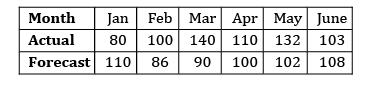Tracking signal value is

Solution: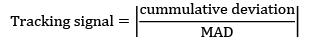n = no. of periods considered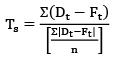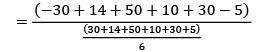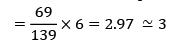QUESTION: 4

The demand for 7 weeks is as follows 90, 93, 96, 99, 102, 105, 108

‘X’ is the forecasted demand for the 8th week using 3 month moving average method. ‘Y’ is the forecasted demand for the 8th week using 4 month moving average method. ‘Z’ is the forecasted demand for the 8th week using regression analysis. What would be the relation between X, Y, Z?

Solution: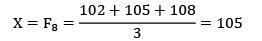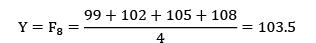Z = F7 = 108 + 3 = 111(Common difference)

∴ Z > X > Y

QUESTION: 5

In a time series forecasting model, the demand for five time periods was 10, 13, 15, 18 and 22. A linear regression fit resulted in an equation F = 6.9 + 2.9 t where ‘F’ is the forecast for ‘t’ period. The mean squared error is ____________

Solution: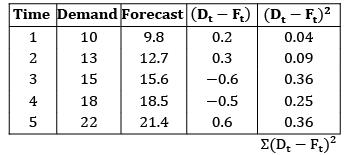= 1.1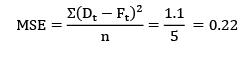QUESTION: 6

Consider the following data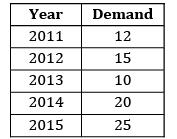Find the forecast for the year 2016 using least squares method.

Solution: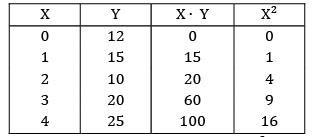ΣX = 10 |ΣY = 82 ΣXY = 195 ΣX2 = 30

Y = a + b ∙ X

ΣY = na + b ∙ ΣX ΣXY = a ∙ ΣX + b ∙ ΣX2

82 = 5a + 10b

195 = 10a + 30b

A = 51/5; b = 31/10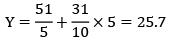QUESTION: 7

Historical data on the sales of cars for the year 2010 to 2016 is given below Find the forecast for the year 2017 using trend line method.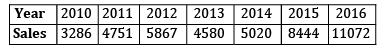Solution: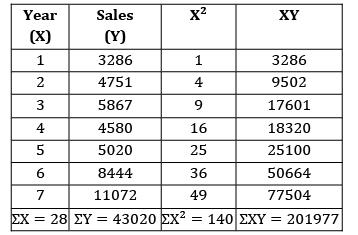Y = a + bX

ΣY = na + b ∙ ΣX → (1)

ΣXY = a ∙ ΣX + b ∙ ΣX2 → (2)

43020 = 7 × a + b × 28

201977 = a × 28 + b × 140

a = 1874.7

b = 1067.75

Y = 1874.7 + 1067.75. X

Y8 = 1874.7 + 1067.75 × 8

Y7 = 10416.7 ≈ 10417

QUESTION: 8

A company forecasts the future demand with “Regression analysis”. The following data is given n = 8; ΣX = 260; ΣY = 420; ΣXY = 14, 900 ΣX2 = 9750; ΣY2 = 23266

The forecast for 10th period is

Solution:

Y = a + bX

ΣY = na + b ∙ ΣX

ΣXY = a ∙ ΣX + b ∙ ΣX2

420 = 8a + b × 260 ⋯ ①

14900 = 260a + b × 9750 ⋯ ②

Solving equations ① and ②

a = 21.3; b = 0.96

Y = 21.3 + 0.96 x

Y10 = 21.3 + 0.96 × 10

= 30.9 ≈ 31

*Answer can only contain numeric values
QUESTION: 9

A company is introducing new product.It contains three alternatives to make the product with the following costs.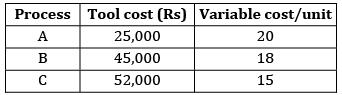The forecasted demand estimates are as follows: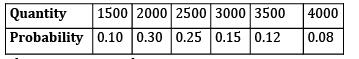The minimum total cost is ____________

Solution: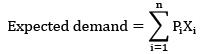= 1500 × 0.10 + 2000 × 0.30 + 2500 × 0.25+3000 × 0.15 + 3500 × 0.12 + 4000 × 0.08

Q = 2565

Total cost = FC + VC × Q

TC)A = 25,000 + 20 × 2565

Rs. 76,300

TC)B = 45,000 + 18 × 2565

= Rs. 91,170

TC)C = 52,000 + 15 × 2565

= Rs. 90,475

Min cost = 76, 300

QUESTION: 10

A company has forecasted the demand value for the month of June as 150 units. The smoothening constant is given as 0.2. The demand values for the month of June, July and August are constant and is given as 140 units. Determine the mean forecast error

Solution:

FJune = 150 units

DJune = 140 units, DJuly = 140

DAug = 140

FJuly = FJune + α(DJune − FJune )

= 150 + 0.2(140 − 150)

= 148 units.

FAug = FJuly + α(DJuly − FJuly )

= 148 + 0.2(140 − 148)

FAug = 146.4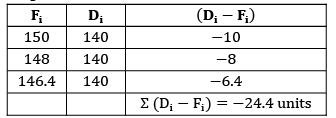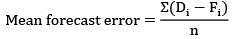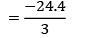= −8.13 units